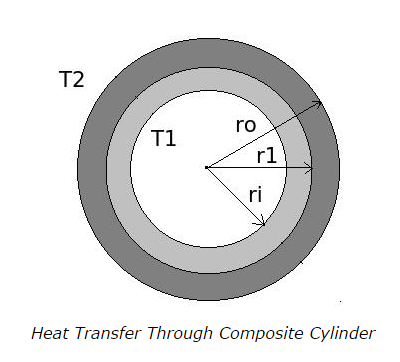Q1. What is the formula for the thermal resistance (R) of a hollow cylinder of internal radius Ri, outer radius Ro and the length l ? Thermal conductivity of the cylinder material is k. The heat flows from inner side to outer side.

a. R = ln (Ro / Ri) / 2πkl
b. R = ln (Ri / Ro) / 2πkl
c. R = (Ro + Ri) / 2πkl
d. R = (RoRi) / 2πkl

ANSWER: a. R = ln (Ro / Ri) / 2πkl

Q2. A hollow cylinder of length of 1m, inner radius (Ri) 0.01m and outer radius (Ro) 0.05m has a thermal conductivity (k) 20 W/mK. The inside surface temperature is at 500K. What is the outside surface temperature of the cylinder when the rate of radial heat transfer is 3.13 kW?

a. 400 K
b. 360 K
c. 460 K
d. insufficient data

Q3. As the radius increases in hollow cylinder the temperature

a. also increases
b. decreases
c. it depends upon the direction of heat flow
d. unpredictable

ANSWER: c. it depends upon the direction of heat flow

Q4. For the composite cylinder as shown in figure, the thermal resistances of every layer from inside to outside of the cylinder are given as follows,

R1 = ln (r1 / ri) / 2πk1l
R1 = ln (ro / r1) / 2πk2l

What will be the total thermal resistance of the composite cylinder?a. R = R1 + R2
b. R = R1R2 / (R1 + R2)
c. R = R1 / (R1 + R2)
d. R = R2 / (R1 + R2)